# 【R语言入门】R语言环境搭建

## 说明

R 语言是一个功能十分强大的工具，几乎绝大多数的数据分析工作都可以在 R 中完成，并且拥有很极强的绘图功能支持，能让你手中的数据以各种姿势进行可视化呈现，而且支持 WindowsMac OSLinux 系统，而且使用起来也比较简单方便。

## R 下载与安装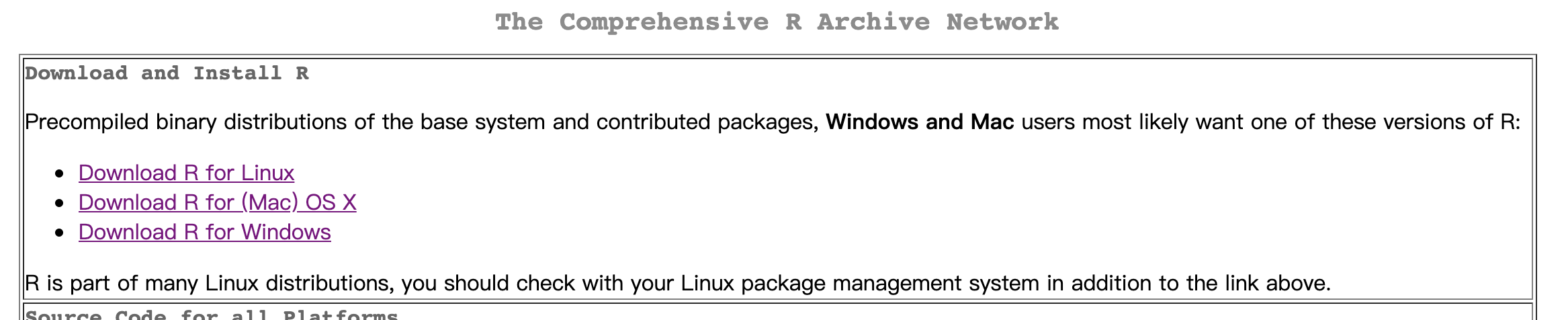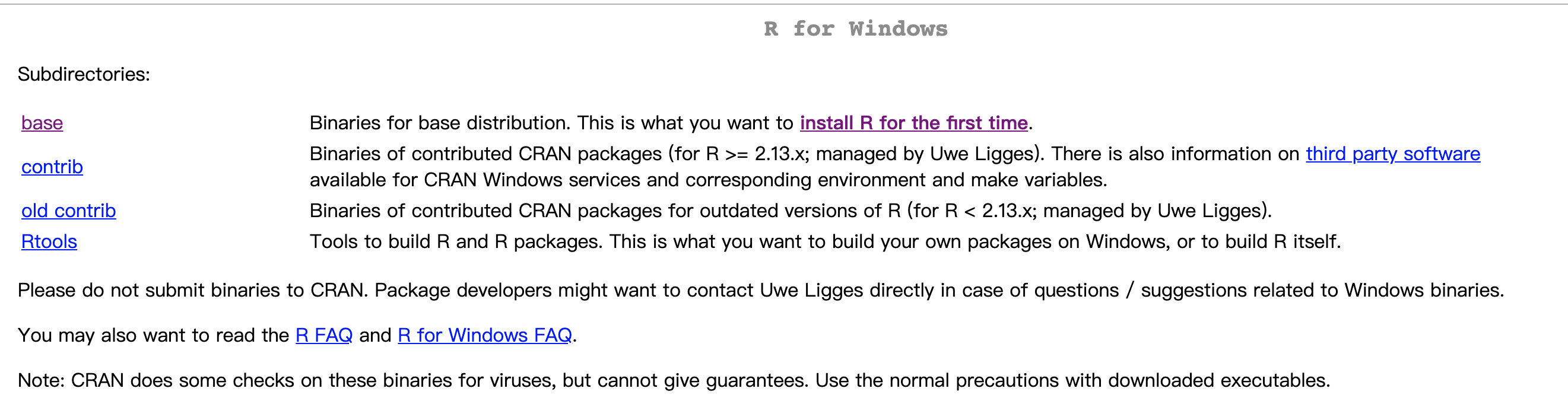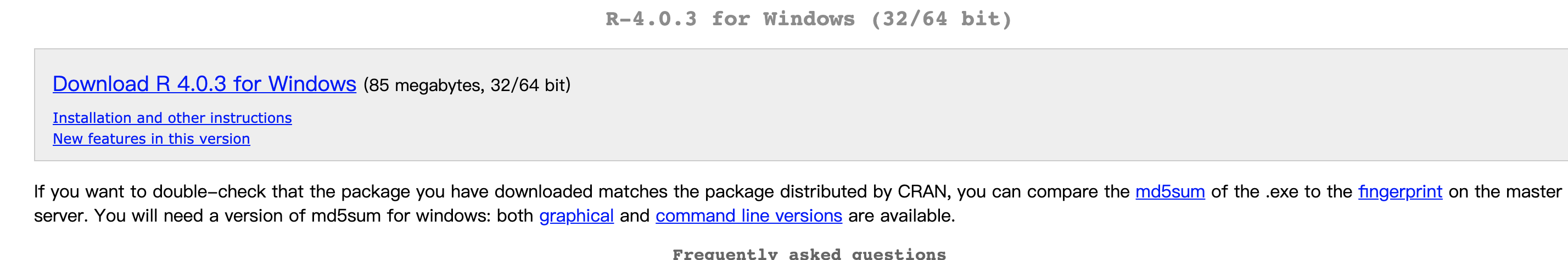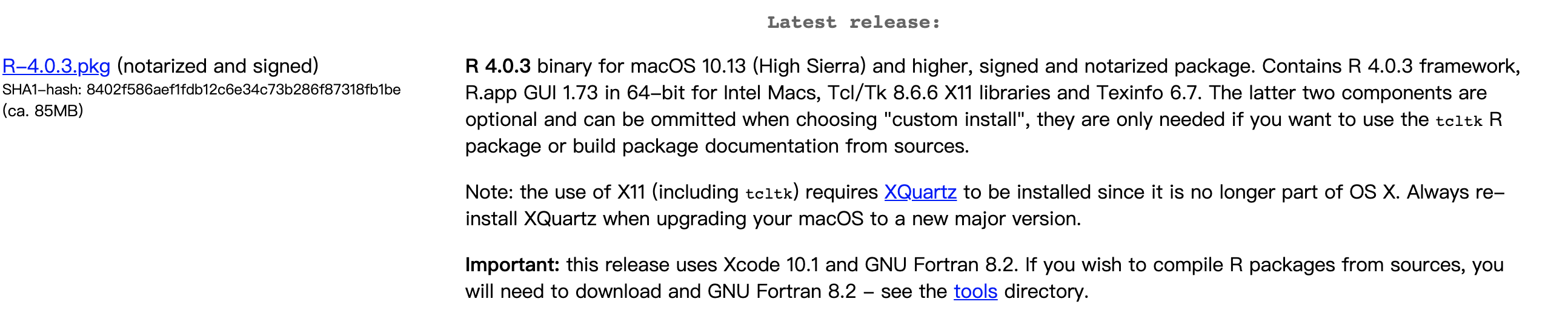## R studio 下载与安装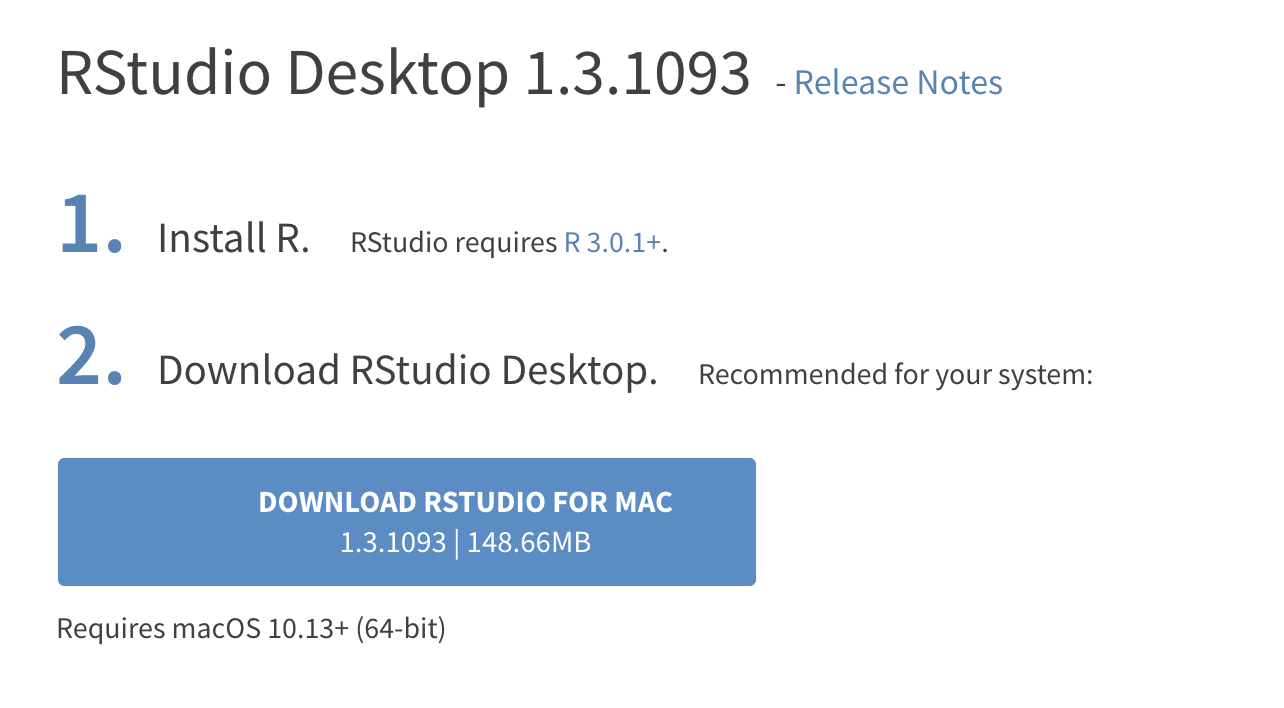## R 语言简单实例

R version 4.0.3 (2020-10-10) -- "Bunny-Wunnies Freak Out"
Copyright (C) 2020 The R Foundation for Statistical Computing
Platform: x86_64-apple-darwin17.0 (64-bit)

R是自由软件，不带任何担保。

R是个合作计划，有许多人为之做出了贡献.consule 面板中输入：example(plot)，轻轻敲击几次回车，就能看到 plot 函数的一些实例了。

> example(plot)

plot> Speed <- cars$speed plot> Distance <- cars$dist

plot> plot(Speed, Distance, panel.first = grid(8, 8),
plot+      pch = 0, cex = 1.2, col = "blue")

plot> plot(Speed, Distance,
plot+      panel.first = lines(stats::lowess(Speed, Distance), lty = "dashed"),
plot+      pch = 0, cex = 1.2, col = "blue")

plot> ## Show the different plot types
plot> x <- 0:12

plot> y <- sin(pi/5 * x)

plot> op <- par(mfrow = c(3,3), mar = .1+ c(2,2,3,1))

plot> for (tp in c("p","l","b",  "c","o","h",  "s","S","n")) {
plot+    plot(y ~ x, type = tp, main = paste0("plot(*, type = \"", tp, "\")"))
plot+    if(tp == "S") {
plot+       lines(x, y, type = "s", col = "red", lty = 2)
plot+       mtext("lines(*, type = \"s\", ...)", col = "red", cex = 0.8)
plot+    }
plot+ }

plot> par(op)

plot> ##--- Log-Log Plot  with  custom axes
plot> lx <- seq(1, 5, length = 41)

plot> yl <- expression(e^{-frac(1,2) * {log(x)}^2})

plot> y <- exp(-.5*lx^2)

plot> op <- par(mfrow = c(2,1), mar = par("mar")-c(1,0,2,0), mgp = c(2, .7, 0))

plot> plot(10^lx, y, log = "xy", type = "l", col = "purple",
plot+      main = "Log-Log plot", ylab = yl, xlab = "x")

plot> plot(10^lx, y, log = "xy", type = "o", pch = ".", col = "forestgreen",
plot+      main = "Log-Log plot with custom axes", ylab = yl, xlab = "x",
plot+      axes = FALSE, frame.plot = TRUE)

plot> my.at <- 10^(1:5)

plot> axis(1, at = my.at, labels = formatC(my.at, format = "fg"))

plot> e.y <- -5:-1 ; at.y <- 10^e.y

plot> axis(2, at = at.y, col.axis = "red", las = 1,
plot+      labels = as.expression(lapply(e.y, function(E) bquote(10^.(E)))))

plot> par(op)
>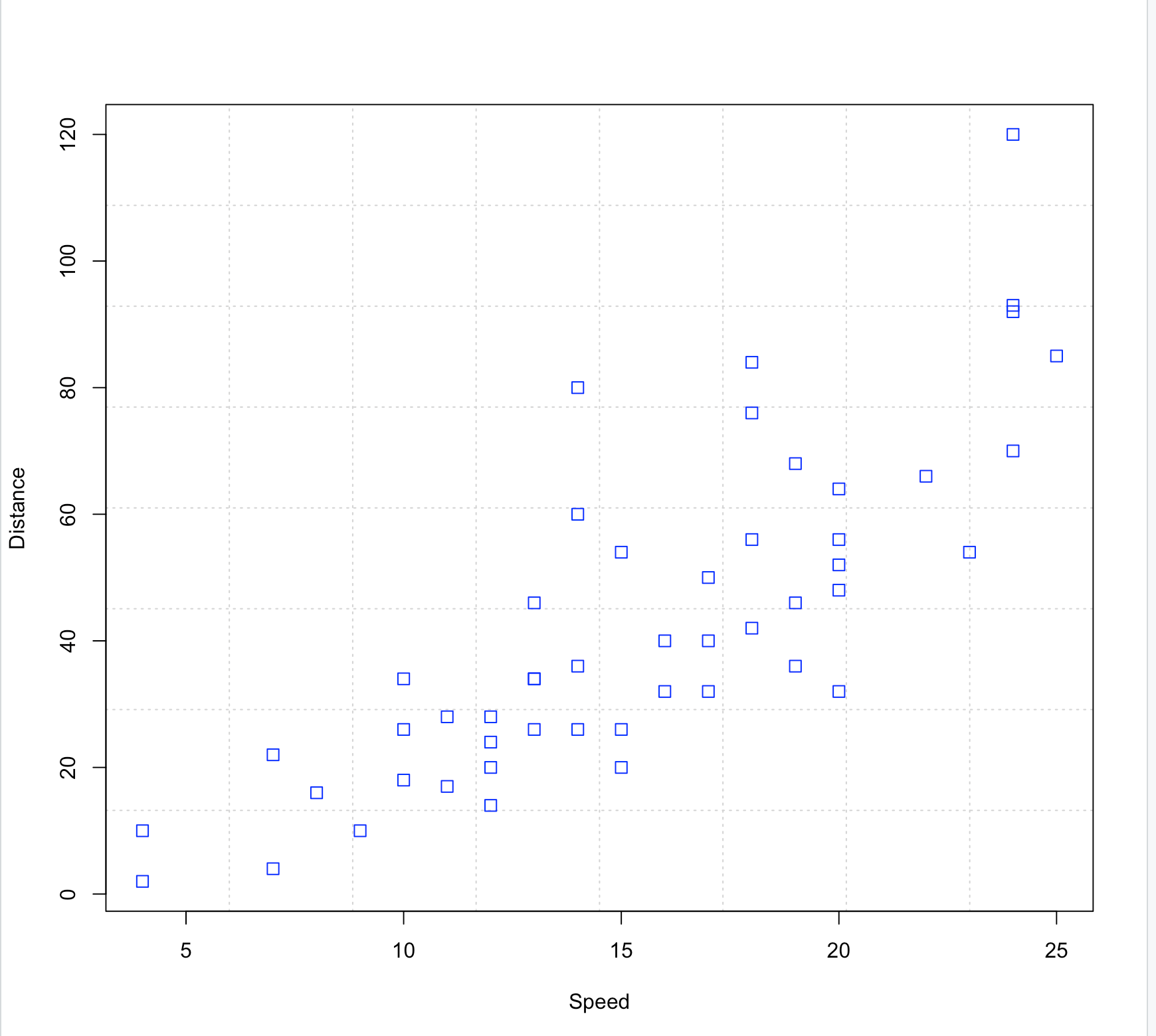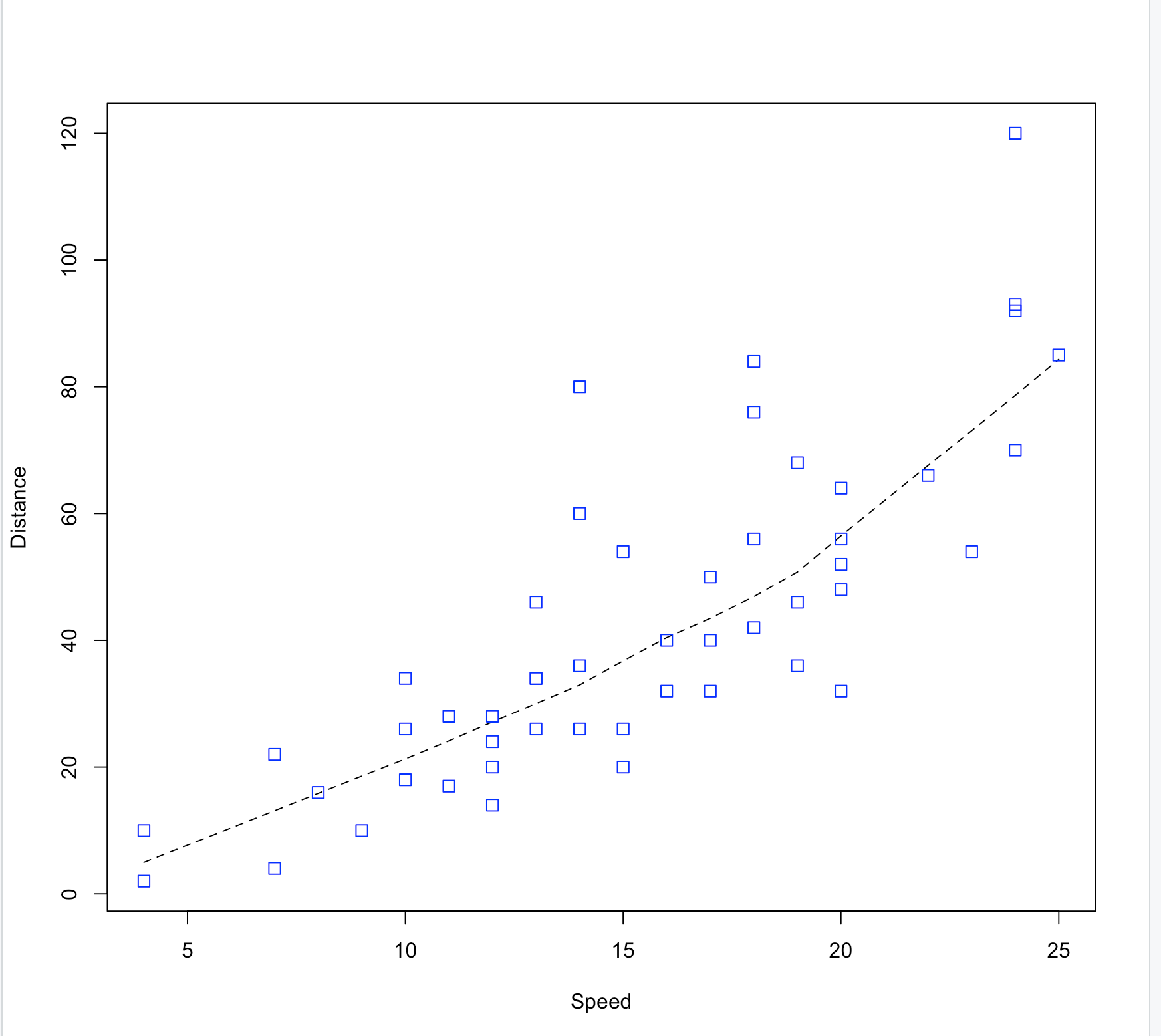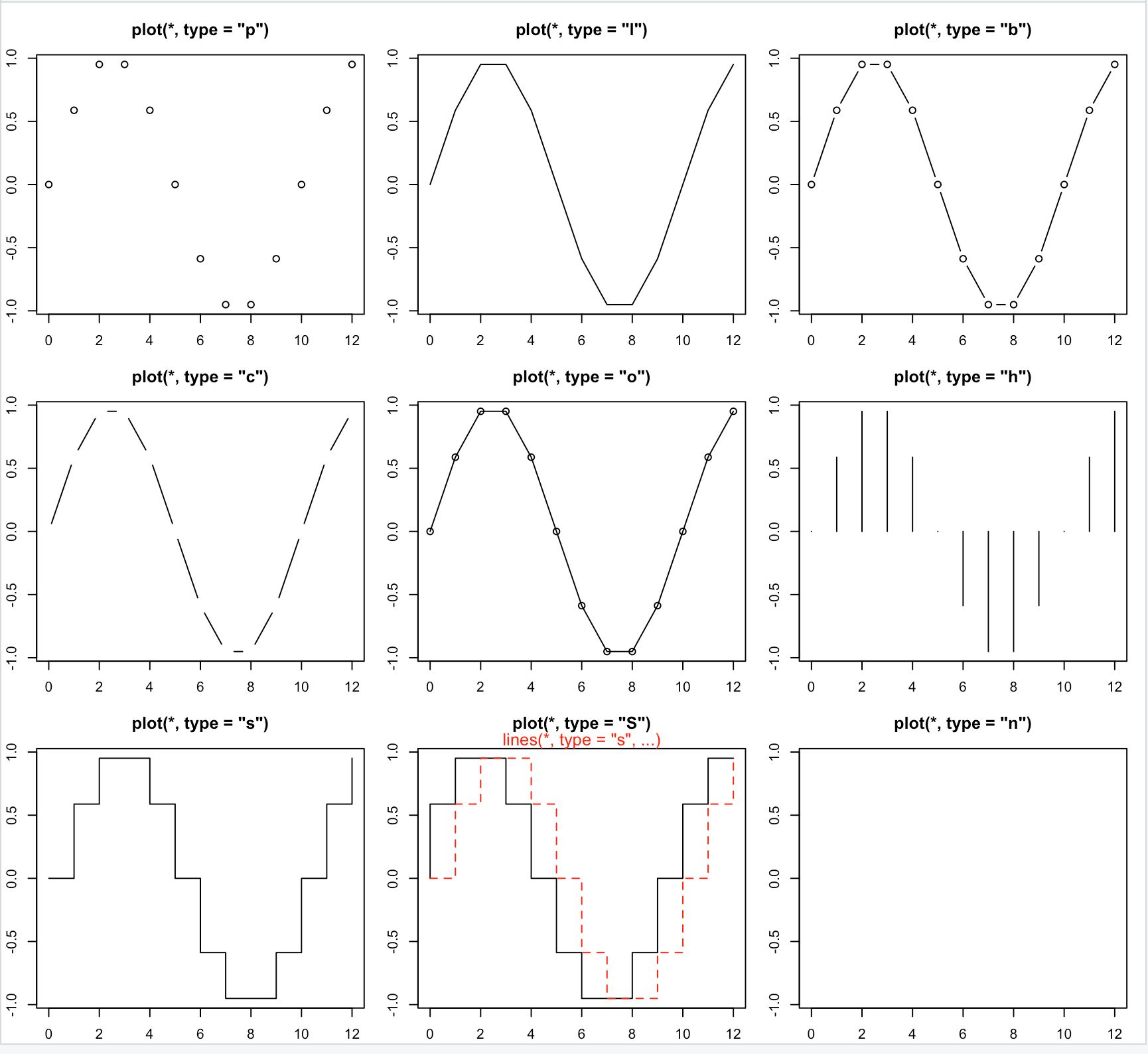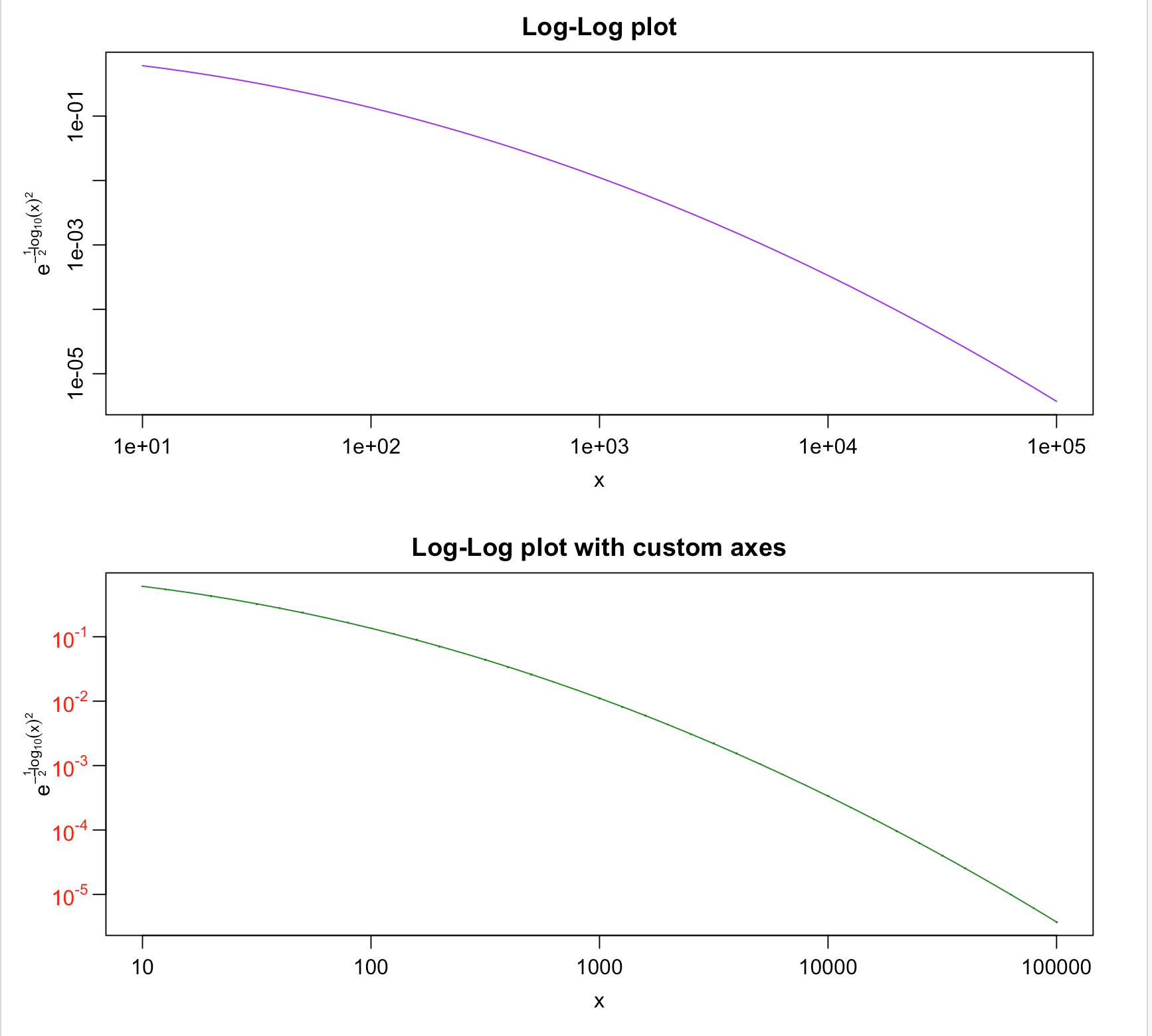## 小结posted @ 2020-11-28 08:16  弗兰克的猫  阅读(888)  评论(0编辑  收藏  举报